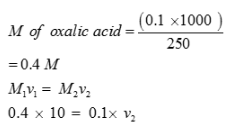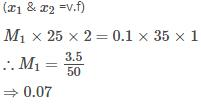Courses

# Test: Solutions- 1

## 25 Questions MCQ Test Chemistry for JEE | Test: Solutions- 1

Description
This mock test of Test: Solutions- 1 for JEE helps you for every JEE entrance exam. This contains 25 Multiple Choice Questions for JEE Test: Solutions- 1 (mcq) to study with solutions a complete question bank. The solved questions answers in this Test: Solutions- 1 quiz give you a good mix of easy questions and tough questions. JEE students definitely take this Test: Solutions- 1 exercise for a better result in the exam. You can find other Test: Solutions- 1 extra questions, long questions & short questions for JEE on EduRev as well by searching above.
QUESTION: 1

### Solubility of gas decreases with

Solution:

For Gasessolubility decreases as temperature increases. The physical reason for this is that when most gases dissolve in solution, the process is exothermic. This means that heat is released as the gas dissolves.

QUESTION: 2

### Solubility of which gas is maximum in water?

Solution:

NHforms hydrogen Bonding with water.

QUESTION: 3

### Molality is preferred over molarity in handling solutions in chemistry laboratory because

Solution:

Molarity is number of moles per unit volume of the solution and molality is number of moles per unit mass of solvent. Volume is temperature dependent where as mass is constant at all temperatures. So, molality remains constant but molarity changes with temperature. Hence , molality is preferred over molarity.

QUESTION: 4

Aquatic animals are more comfortable in cold water rather that warm water because

Solution:

The amount of dissolved oxygen in water decreases with rise in the water's temperature. Cold water has more dissolved oxygen per unit area than warm water. This the reason why aquatic animals are more comfortable in cold water than warm water.

QUESTION: 5

The molal depression constant depends upon

Solution:

Molal depression constant : It is defined as the depression in freezing point when the molality of the solution is unity, i.e., one mole of the solute is dissolved in 1000 gram or 1 kilogram of the solvent.

QUESTION: 6

In the depression of freezing point experiment, it is found that

Solution:

Addition of non volatile solute decreases the vapour pressure.

QUESTION: 7

If the temperature of an aqueous solution increases, it will cause

Solution:

As temperature increases volume also increases and so Molarity decrease.

QUESTION: 8

Solution is defined as

Solution:

Solution is Homogeneous mixture of Solute and Solvent.

QUESTION: 9

Amalgam of mercury with sodium is an example of

Solution:

Solute is mercury( liquid) and solvent is sodium.

QUESTION: 10

Which among the following is miscible in each other?

Solution:

Methanol and Water both are Polar. There is intermolecular Hydrogen Bonding interaction.

QUESTION: 11

Which among the following is an example of liquid in solid?

Solution:

Hydrated salts have water molecule of hydration as solute.

QUESTION: 12

Which among the following is an example of a solid solution in which the solute is a gas?

Solution:

Pumice stone is example of gas in Solid.

QUESTION: 13

The type of intermolecular interaction present in n-Hexane and n-Octane is

Solution:

Both are Non Polar.

QUESTION: 14

Mathematical expression relating molarity and molality is

Solution:

m = moles of solute / mass of solvent. M = moles of solute / volume of solution.

QUESTION: 15

The tanks used by divers are filled with air diluted with

Solution:

Size of Helium is small so does not causes “Bents” to divers when the dive back to surface.

QUESTION: 16

Ethylene glycol is added to water as antifreeze. It will

Solution:

Adding ethylene glycol to water reduces the freezing point of waterWater freezes at temperatures much less than 0 degree Celsius when ethylene glycol is added to it. It is used as an anti-freeze

QUESTION: 17

Which of the following solutions of H2SO4 is more concentrated?

Solution:

The correct answer is option A
1 M H2SO4 solution is more concentrated than 1 N acid solution. Actually 1M solution of acid is prepared by dissolving 98 g of the acid (1 gram mole) per litre of the solution while 1 N solution of acid is prepared by dissolving 49 g of the acid (1 gram equivalent) in the same volume of the solution.

QUESTION: 18

An aqueous solution of methanol and water has vapour pressure

Solution:

They show positive deviation.

QUESTION: 19

Which has highest freezing point at 1 atm?

Solution:

Sugar solution has i=1 so ΔTf minimum so Tf will be maximum.

QUESTION: 20

A solution showing a large positive deviation from ideal behaviour has

Solution:

A solution showing a large positive deviation from ideal behaviour shows lower boiling point than both the components.

QUESTION: 21

Solution of hydrogen in palladium is an example of

Solution:

Hydrogen (solute , gas ) and solvent is palladium (solid).

QUESTION: 22

In which unit, the concentration of solution remains independent of temperature

Solution:

It is independent of volume hence independent of Temperature.

QUESTION: 23

6.3 g oxalic acid is used to make 250 mL aqueous solution .The volume of 0.1N NaOH solution required to neutralize completely 10 mL of this solution is

Solution:v= 40 ml

QUESTION: 24

The depression in freezing point for 1M urea, 1 M glucose and 1 M NaCl are in the ratio of

Solution:

NaCl has i = 2 while urea and glucose will have i = 1

QUESTION: 25

25ml of a solution of barium hydroxide titration with 0.1molar solution of hydro-chloric acid gave a titre value of 35ml.The molarity of barium hydroxide is

Solution:

Ba(OH)2 + 2HCI → BaCl2 + 2H2O

M1V1X1 →  M2V2X2